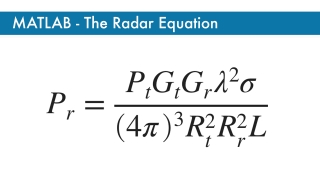# Matlab: The Radar EquationBeginner
Article / Blog
Theory

This Mathworks page explains the parameters of the radar range equation. The point target radar range equation estimates the power at the input to the receiver for a target of a given radar cross section at a specified range. In this equation, the signal model is assumed to be deterministic.

This resource is included in the following topics and journeys: